# Trigonometry 23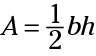where A is the area, b is the length of the triangle’s base, and h is the height of the triangle drawn perpendicular to that base.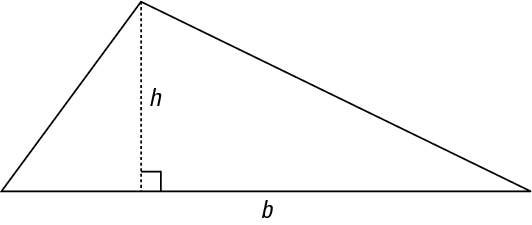This area formula works fine if you can get the measure of the base and the height, and if you can be sure that you’ve measured a height that’s perpendicular to the side of the triangle. But what if you have a triangular yard — a big triangular yard — and have no way of measuring some perpendicular segment to one of the sides?

One alternative is to use Heron’s Formula, which uses the measures of all three sides. The other alternative, of course, is to use trigonometry — or, at least, a formula with an angle measure in it.

To measure that angle, you can be very sophisticated and get a surveying apparatus, or if you’ve got a protractor handy, you can do a decent estimate by extending the sides at an angle for a bit and eyeballing the angle size.

The trig formula for finding the area of a triangle is;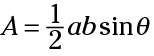where a and b are two sides of the triangle and theta is the angle formed between those two sides. You don’t need the measure of the third side at all, and you certainly don’t need a perpendicular side.

Take a look at the triangle shown, with sides a and b and the angle between them.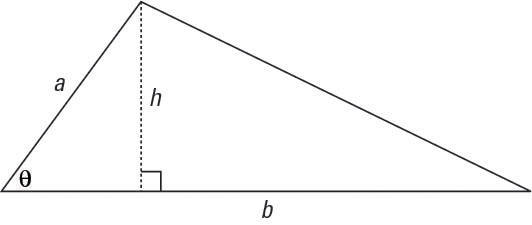Then look at the smaller triangle to the left. (Because the height is drawn perpendicular to the base, the sides and height form a right triangle.) The acute angle has a sine equivalent to the following: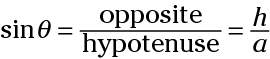If you solve that equation for h by multiplying each side by a, you get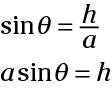Replace the h in the traditional formula with its equivalent from the preceding equation, and you get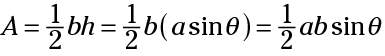Check out how this formula works in an actual problem. The triangle shows the measures of two of its sides and the angle between them.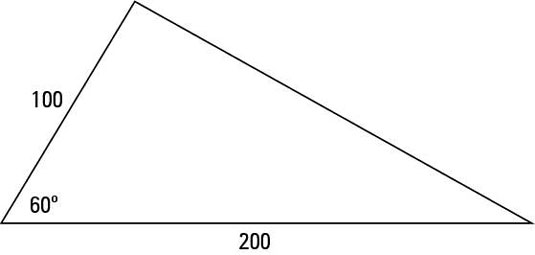To find the area of the triangle:

• Use the formula
•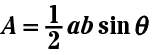• inserting the values that you know.
•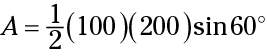• Solve for the value of the area.
•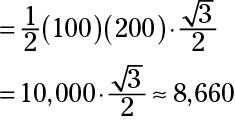Example:

BC = 12, AC = 7, m = 820

Find its area.

Express the area rounded to three decimal places.

x

Explanation:

A = 1/2 ab sin C

A = 1/2 X 12 X 7 sin 82°

A = 42(0.9903)

A = 41.6

x

Practice Problem #1)

In ΔPQR, PQ = 27, PR = 18, and m< P = 570

Find the area of ΔPQR to the nearest tenth of a square unit.

x

Explanation #1

A = 1/2 ab sin P

A = 1/2 x 27 x 18 x sin 570

A = 243(0.8387)

A = 203.8

x

Practice Problem #2)

In an isoscelesΔ, the two equal sides each measure 22 meters, and they include an angle of 480. Find the area of the isosceles triangle, to the nearest square meter.

x

Explanation #2

A = 1/2 ab sin X

A = 1/2 x 22 x 22 x sin 480

A = 484 (0.7431)

A = 359.7

x

Review

Directions: Solve each of the following problems.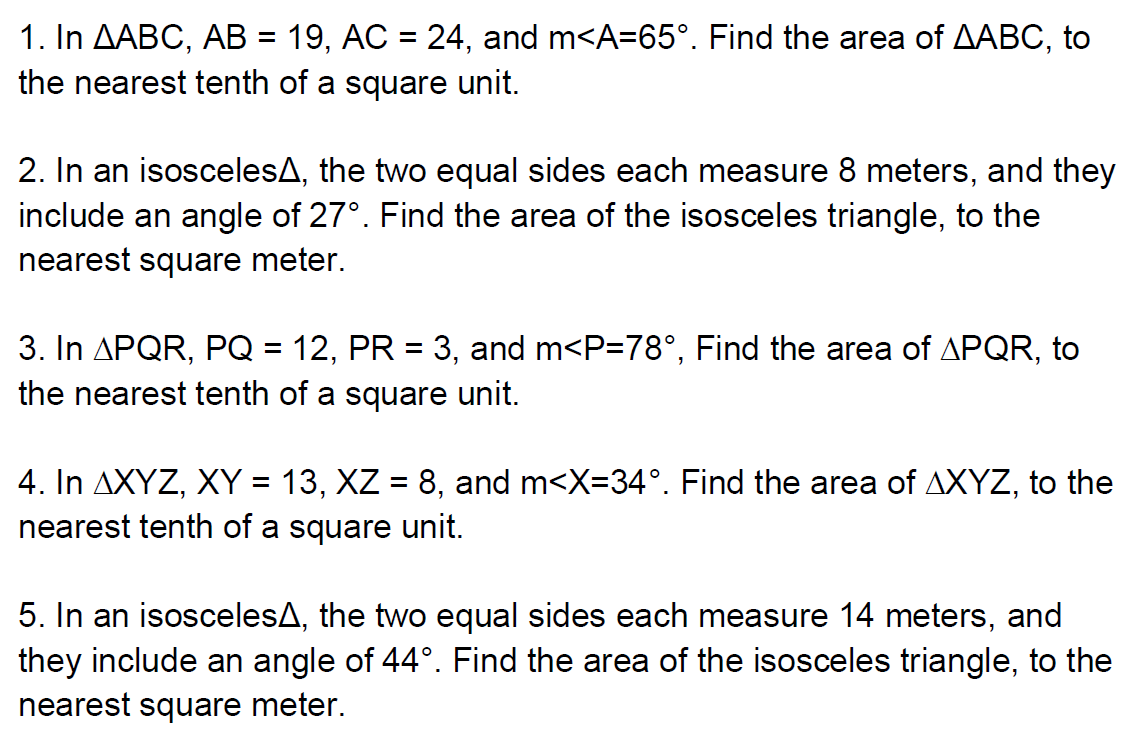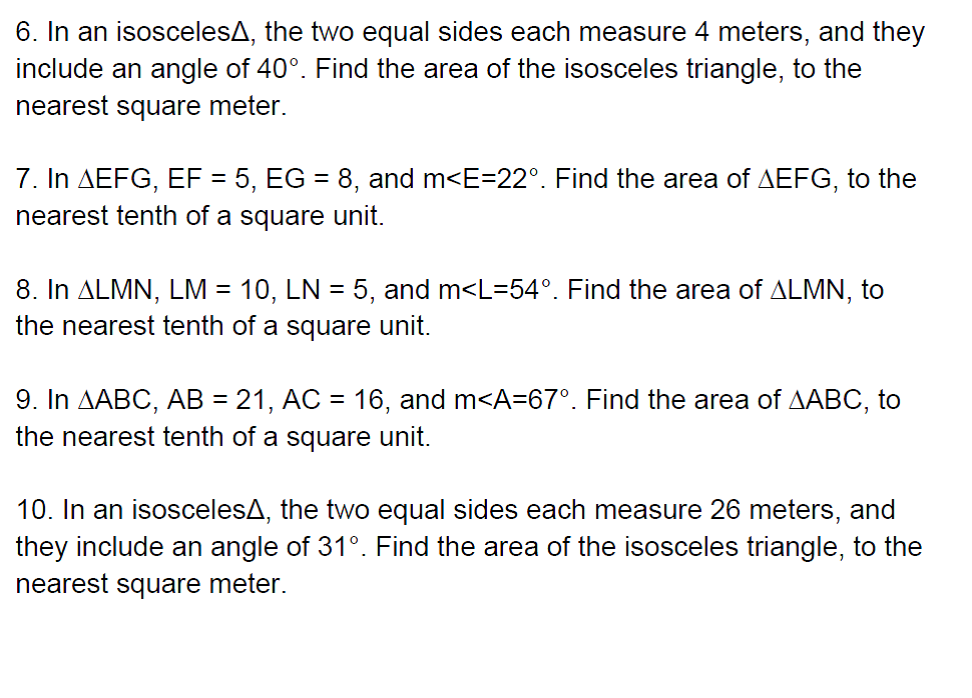#### Looking for this or a Similar Assignment? Click below to Place your Order